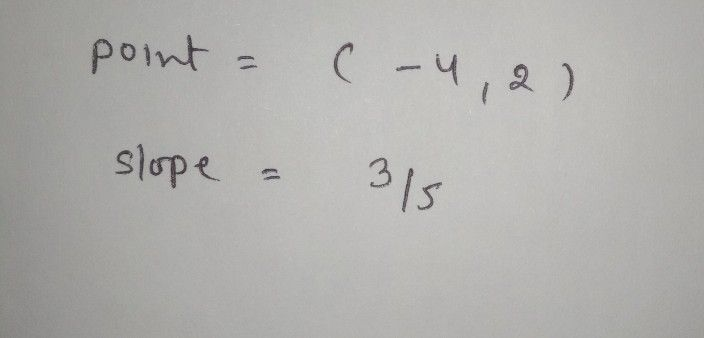Symbol
ProblemQUESTION $2$ Use the following linear equation: $y+4=$ $frac|\left(3\right)\left(53\left(x-2\right)$ Point $=\left($ $S$ Slope $=$
Algebra
Question content
This is from a algebra and trig class .. please make sure hand writing is good
Solution